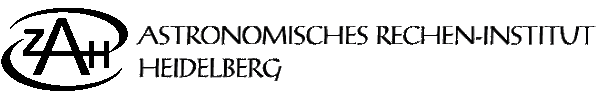ARIPRINT:    Database of publications of the institute

ARI-Heidelberg Mitteilungen Serie B, No. 40

 Author(s): Miyamoto, M. Title: A Class of Disk-like Models for Self-gravitating Stellar Systems Source: Astron. Astrophys. 30, 441-454 Year: 1974 Abstract: A construction scheme is proposed for disk-like models of self-gravitating steady-state stellar systems by using Fricke's (1952) type of expansion for the velocity distribution function f(E,J) with respect to the energy integral E and the angular momentum integral J. The distribution function is analyzed by decomposing into three parts such as f(E,J)=f1(E)+f2(E,J2)+f3(E,J). In order to avoid negative-valued f(E,J), it is assumed that a given density, the gravitational potential and the partial density, which is derived from f1(E) only , are monotonically decreasing with the distance from the center, and the coefficients of all the terms in f2(E,J2) and f3(E,J) are positive. Properties of each expansion term in f2(E,J2) and the corresponding partial density are investigated; (1) each term in f2(E,J2) attains two maxima, located symmetrically in the velocity space, at an azimuthal velocity smaller than 2-1/2 times the escape velocity, (2) the equi-distribution curves near the two maximum points are approximately ellipses, (3) the axial ratio of the ellipses coincides with the well-known relation between the velocity dispersions and the Oort constants, (4) the skewness of the velocity distribution is indicated by an ellipse with its center at the origin of the velocity space, (5) in a limit of infinitely large exponents of E and J, each term tends to a delta-function with two peaks located symmetrically in the velocity space, (6) the corresponding partial density has one maximum, if the circular velocity of the disk is monotonically increasing with the distance from the center and is smaller than 2-1/2 times the escape velocity. Self-consistent models are possible in the whole range from an extremely "cold" state to an extremely "hot" state of the disk. Preprint issued: Remarks:Full text of the printed paper in the ARIPRINT in the following format:
[Image (GIF)] [GIF in frames]

Letzte Änderung/Updated: 12.10.2001

ariprint@ari.uni-heidelberg.de